Author: Oscar Cronquist Article last updated on October 18, 2019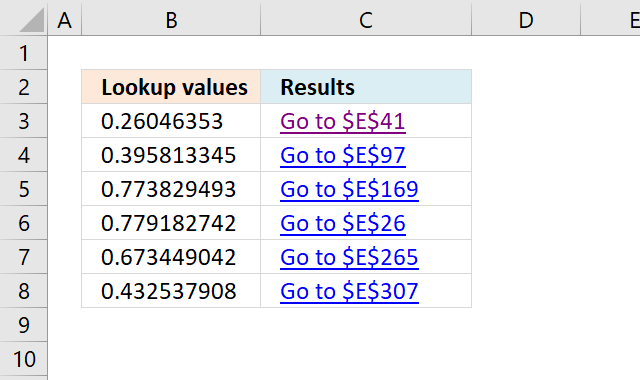Today I'll show you a formula that returns a hyperlink pointing to a location based on a lookup value. When you press with left mouse button on the cell that contains the hyperlink text, Excel takes in an instant to that location in the table.

The formula is dynamic meaning if you change the search value the formula changes the location and the hyperlink text instantly.

Formula in cell C3:

This formula finds only the first instance of the search value, se further down the article for a formula that returns multiple results.

###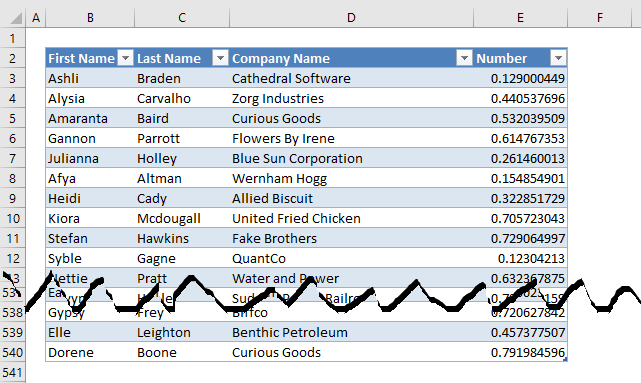The Excel defined Table is located on worksheet "Table", the image above shows some records of that Excel defined Table.

### Explaining formula in cell C3

I recommend you use the "Evaluate Formula" feature in Excel to examine calculations step by step.

Select cell C3, go to tab "Formulas" on the ribbon and press with left mouse button on the "Evaluate Formula" button.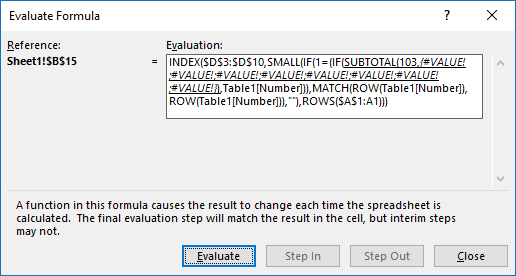(The formula shown in the above image is not the formula used in this article.)

Press with left mouse button on "Evaluate" button to see the next step in the formula calculations.

#### Step 1 - Return the relative position of an item in an array that matches a specified value

The MATCH function returns the relative position of a value in a column.

MATCH(A2, Table1[Number], 0)

becomes

MATCH(0.260463529541505, {0.129000449044708;0.440537695535749;0.532039509437168; ...}, 0)
(Array shortened)

and returns 39.

#### Step 2 - Create a cell reference as text

The ADDRESS function returns a cell reference based on a row and column number. ADDRESS(row_numcolumn_num, [abs_num], [a1], [sheet_text])

becomes

The MATCH function returns a relative position, it does not take into account cells above the Excel Table. We need to determine how many cells are above the Excel Table.

becomes

becomes

The second argument is the column number and is hardcoded into the formula, you must change this to the column number to a number representing the column you search.

and returns \$E\$41

#### Step 3 - Create a shortcut to a cell in sheet Table

becomes

=HYPERLINK("[Find values quickly.xlsx]Table!\$D\$41, "Go to \$D\$41")

###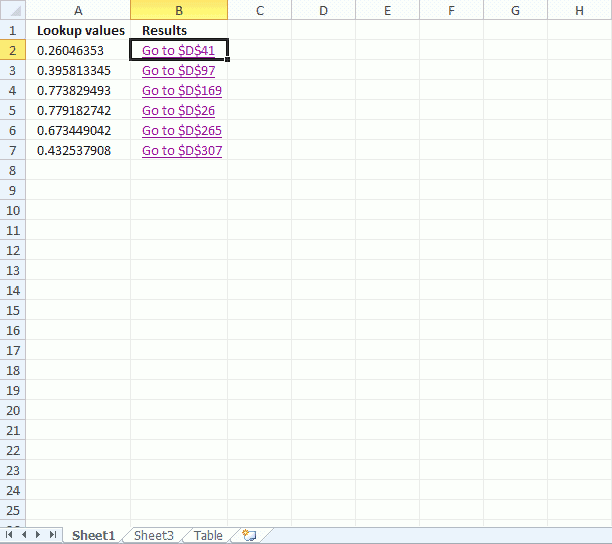The animated image above shows what happens when you press with left mouse button on a hyperlink, note that the lookup value match the number.

### What if there are multiple values matching your lookup value?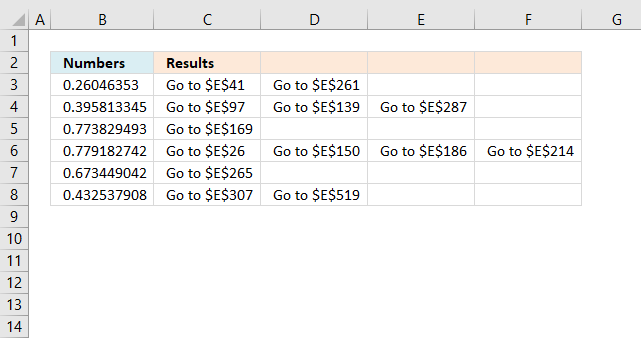Array formula in cell C3:

### How to create an array formula

1. Copy above array formula
2. Select cell B2
3. Press with left mouse button on in formula bar4. Paste array formula
5. Press and hold Ctrl + Shift
6. Press Enter

How to copy array formula

1. Select cell B2
2. Copy cell (Ctrl + c)
3. Select cell range C2:E2
4. Paste (Ctrl + v)
5. Select cell range B2:E2
6. Copy (Ctrl + c)
7. Select cell range B3:E7
8. Paste (Ctrl + v)

### Explaining formula in cell B2

Step 1 - Check if a condition is met and return corresponding row value if TRUE

IF(\$A2=Table1[Number], ROW(Table1[Number]), "")

becomes

IF(0.260463529541505={0.129000449044708;0.440537695535749;0.532039509437168; ...}, {3, 4, 5, ...}, "")

and returns

{""; ""; ""; ""; ""; ""; ""; ""; ""; ""; ""; ""; ""; ""; ""; ""; ""; ""; ""; ""; ""; ""; ""; ""; ""; ""; ""; ""; ""; ""; ""; ""; ""; ""; ""; ""; ""; ""; 41; ... ; ""} (Array shortened)

Step 2 - Return the k-th smallest value

SMALL(arrayk)

SMALL(IF(\$A2=Table1[Number], ROW(Table1[Number]), ""), COLUMNS(\$A\$1:A1))

becomes

SMALL({""; ""; ""; ""; ""; ""; ""; ""; ""; ""; ""; ""; ""; ""; ""; ""; ""; ""; ""; ""; ""; ""; ""; ""; ""; ""; ""; ""; ""; ""; ""; ""; ""; ""; ""; ""; ""; ""; 41; ... ""}, 1)

and returns 41.

Step 3 - Create a shortcut to a cell in sheet Table